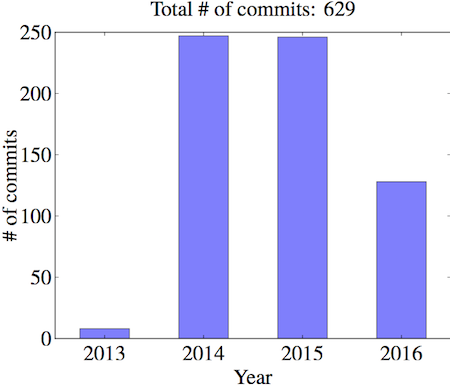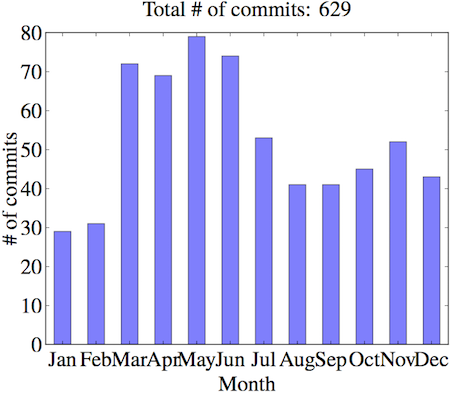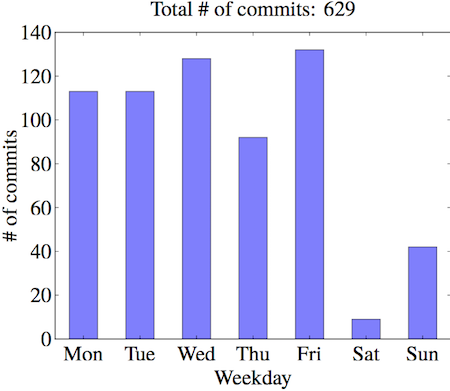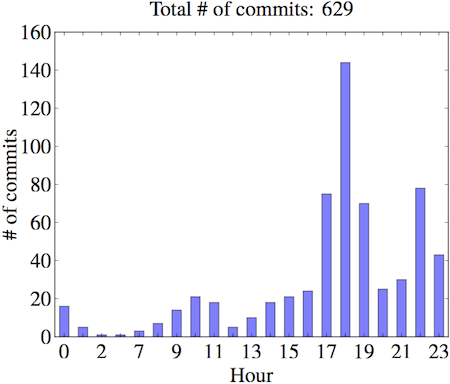# 博士项目的一些“小数据”

11 分钟阅读

### 年### 月### 星期### 小时

1. 主要集中在下班时间（17 点-19 点），记录今天工作结果
2. 其次说明我下班后有时候还工作…睡觉前再来一个 commit 然后第二天继续工作…from homepylab import *
import pandas
from collections import Counter
import subprocess
import os

out = subprocess.check_output(["hg", "log"])

fold = open("old-commits", "r")
f = open("out", "w")
f.writelines(out)
for line in fold:
f.write(line)
f.close()

f = open("out", "r")
weekday = []
month = []
year = []
hour = []
day = []
for line in f:
if line.startswith("date"):
data = line.split()
weekday.append(data)
month.append(data)
year.append(data)
hour.append(int(data.split(":")))
day.append(int(data))

for item in ["weekday", "month", "year", "hour", "day"]:

figure()
letter_counts = Counter(eval(item))
df = pandas.DataFrame.from_dict(letter_counts, orient="index")
if item == "weekday":
index = [u'Mon', u'Tue', u'Wed', u'Thu', u'Fri', u'Sat', u'Sun']
df = df.reindex(index)
elif item == "month":
index = [u'Jan', u'Feb', u'Mar', u'Apr', u'May', u'Jun', u'Jul', u'Aug', u'Sep', u'Oct', u'Nov', u'Dec']
df = df.reindex(index)
elif item == "year":
index = ["2013", "2014", "2015", "2016"]
df = df.reindex(index)

fig = df.plot(kind="bar", legend=False, alpha=0.5, rot=0)

ylabel(r"\# of commits")
xlabel(item.capitalize())
title(r"Total \# of commits: %d" %(len(eval(item)))).set_y(1.04)
savefig("nbcommits_%s.ipe" %(item))

os.remove("out")